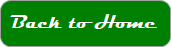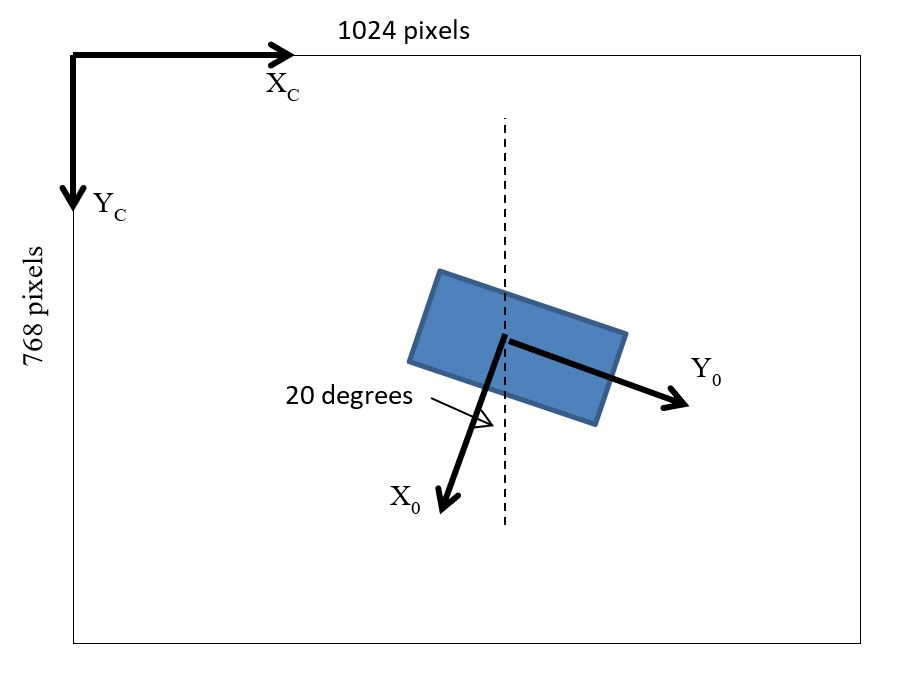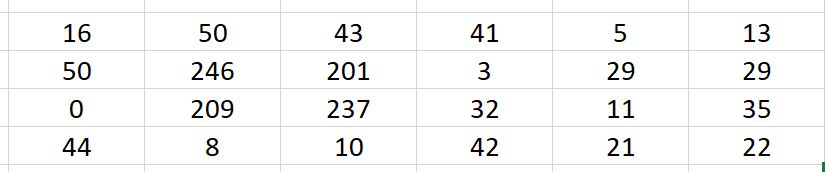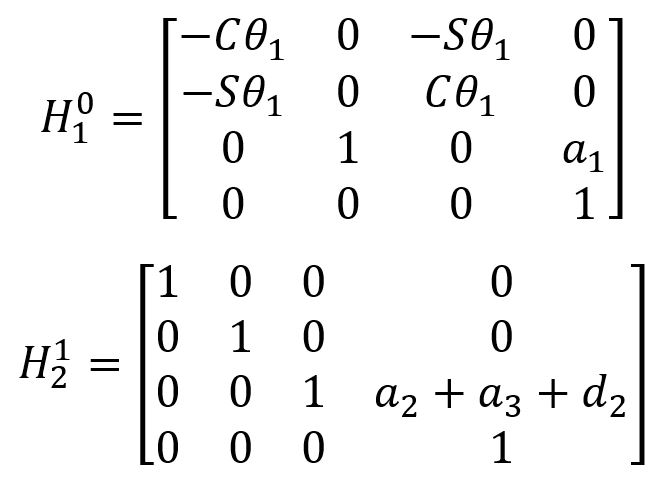Robotics 1
Checkpoint 2: Sensors and VisionExample Question 2:  Suppose you want to use the encoder from Question 1 to measure motor speed.  When the motor speed is set to 20 rpm, how many counts will be read per second?
Example Question 3:  Now suppose that we set the PSoC counter resolution to 1x instead.  When the motor is the same 20 rpm, how many counts will be read per second?
Example Question 4:  Suppose you set the counter resolution to 2x and you want to display the value of the variable 'rotational angle' in units of degrees to the LCD screen.  Find an equation to calculate rotational angle from the number of counts read from the encoder.
Example Question 5: Suppose I want to use this same encoder to measure motor speed, and I know that the fastest speed my motor can turn is 1000 rpm.  Suppose I know that the fastest I can count 'counts' from an encoder is 1 count per millisecond.  Will I be able to measure this motor speed?  If so, which counter resolution (1x, 2x, or 4x) should I use?  At the 4x resolution, what is the fastest motor speed I can measure?
Example Question 6:  What is the largest number that can be represented with 10 bits?  What is the number of different numbers that can be represented with 10 bits?
Example Question 7: Suppose I have a potentiometer that can turn 270 degrees while the voltage at the wiper varies between 0V and 5V.  Suppose I am reading the voltage on an ADC pin with a 10-bit resolution.  What is the smallest change in angle that I can detect?
Example Question 8: For question 7, when the angle is exactly 180 degrees, what will be the bit string stored by the ADC converter?
Example Question 1: You have bought an incremental quadrature encoder with 100ppr (pulses per revolution).  You have attached this encoder to the shaft of a motor that has no gearbox, and have hooked up the encoder to your PSoC at a 4x counter resolution so that you get one count for each pulse.  What is the smallest angle change (in units of degrees) that you can detect?
Congratulations!  You've made it all the way through our second unit: Sensors and Vision.  Before we move on, you will need to take a test to make sure that all this information has sunk in.  Here are some example problems like the ones you will see on the test in class.  On our review day (the class day before the test), I'll go through these problems live on the board.  So, it's a good idea to try to solve the problems on your own before coming to class.
Example Question 9: For question 7, suppose the bit string read by the converter is 1001010111.  What is the RANGE of angles that the potentiometer might have?
Example Question 10: Suppose I take one color image at 640x480 resolution with a 10-bit depth and one grayscale image at 1080x720 resolution with 8-bit depth.  How many NUMBERS are contained in the matrices for each image?  How many BITS of information are contained in the matrices for each image?Example Question 11: Name two conditions that will cause background subtraction to fail to find an object, and name one condition that will cause color subtraction to fail to find an object.Example Question 12: Suppose I have a grayscale image as shown here.  Where is the object located, in units of pixels, in the X and Y directions?  Use the 'center of mass' approach to solve this problem, and give your answer in units of 'pixels'.
Example Question 13: You are using machine vision to do automated inspection of parts on an assembly line, and you have found an object as shown here.  Suppose you know that the field of view of the camera is 20 cm in the Yc direction, and suppose you have detected the center of the object to be at 600 pixels in the Xc direction and 400 pixels in the Yc direction.  Find the homogeneous transformation matrix H0_C.  When you are finding the displacement vector part of the homogeneous transformation matrix, use the provided ruler.Example Question 14:  For Question 13, suppose you have found another object located at 100 pixels in the Yc direction and 100 pixels in the Xc direction.  Where is the object located, in units of centimeters, in the 0 frame?  Use your homogeneous transformation matrix from Question 13 to solve the problem.Graduates-Only Example Question 1:  Shown here is a kinematic diagram that I have labeled, and all of the homogeneous transformation matrices for the manipulator.  Find the Jacobian matrix.
Graduates-Only Example Question 2:  Shown here is a Jacobian matrix which I have found.  Do some analysis of the velocities of this manipulator: which velocities will always be zero?  Which velocities are controlled by only one joint?
Watch the solutions to the undergraduate example questions (there is no audio in this video):